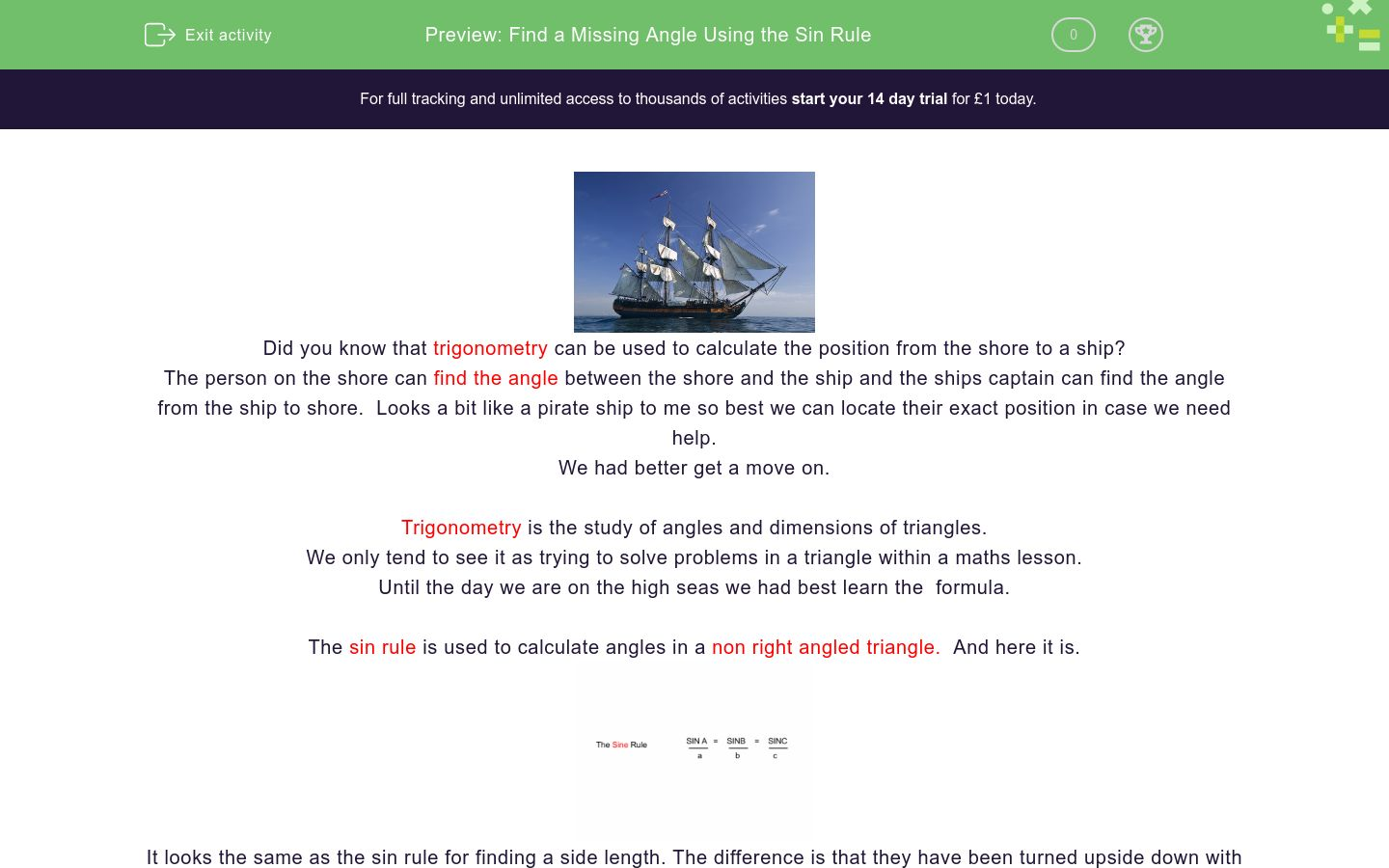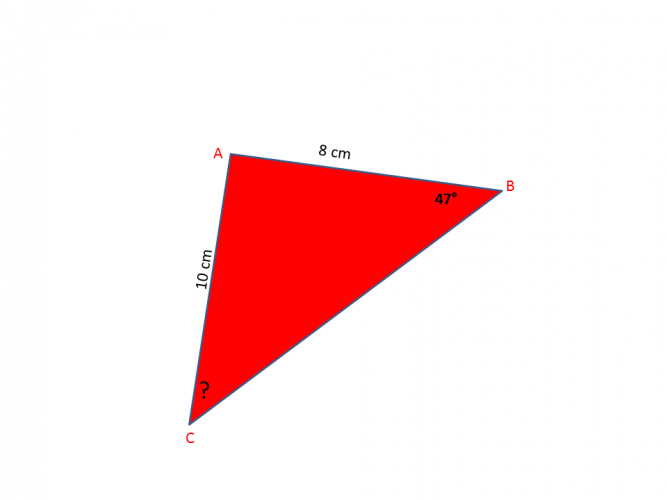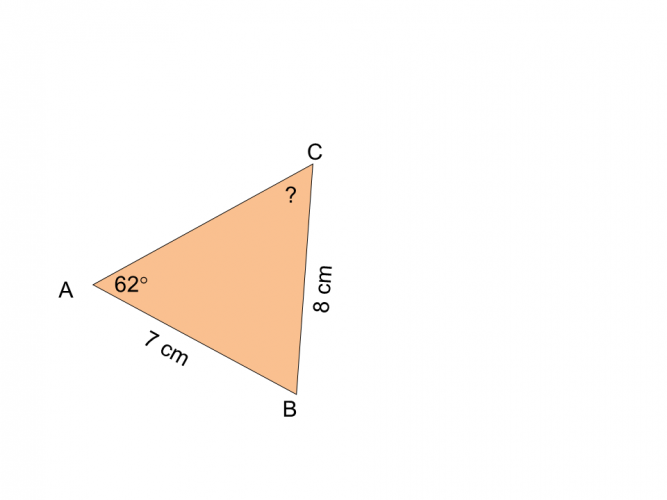# Find a Missing Angle Using the Sin Rule

In this worksheet, students will apply the sin rule to find out angles within a non-right angled triangleKey stage:  KS 4

GCSE Subjects:   Maths

GCSE Boards:   AQA, Eduqas, Pearson Edexcel, OCR

Curriculum topic:   Geometry and Measures, Mensuration

Curriculum subtopic:   Mensuration and Calculation, Triangle Mensuration

Difficulty level:### QUESTION 1 of 10Did you know that trigonometry can be used to calculate the position from the shore to a ship?

The person on the shore can find the angle between the shore and the ship and the ships captain can find the angle from the ship to shore.  Looks a bit like a pirate ship to me so best we can locate their exact position in case we need help.

We had better get a move on.

Trigonometry is the study of angles and dimensions of triangles.

We only tend to see it as trying to solve problems in a triangle within a maths lesson.

Until the day we are on the high seas we had best learn the  formula.

The sin rule is used to calculate angles in a non right angled triangle.  And here it is.It looks the same as the sin rule for finding a side length. The difference is that they have been turned upside down with the angles (SIN) being on the top.

You will need a scientific calculator in order to get the sin-1 button. Make sure it is set to degrees.

Example 1The triangle is labelled in capital letters, with the opposite side to them labelled in lower case letters.

Getting the opposite side labelled correctly is key here.

We want angle b.

The only other information we have is angle A, side a and side b.

Our formula therefore is      SIN A          =    SIN B

a                     b

Substitute in what we know  SIN 98        =   SIN B

8.3                   6Now for the geeky bit

We need to get  SIN B on its own, so we need to rearrange the formula.  We move 6 up to SIN98 to get:

6 x sin98  = 5.941...... do this first to get an answer THEN divide by 8.3 = 0.7158

Now because we are looking for an angle we need the sin-1 button. (Press shift then sin on your calculator)

and viola ....45.7° (to 3 significant figures)

NOTE: If you try to put it all into the calculator at once the rules of bidmas aren't followed and your answer will be incorrect.

Example 2We need SIN B   =  SIN C

b            c

Substitute in what we know  SIN 37      =  SIN C

6                 5

Bring 5 up to up to sin37  to give us 5 x sin37  =  3.009 ÷ 6 = 0.501

sin-1 = 30.1°( to 3 significant figures)

Let give it a try..Find the angle ACB.

Answer has been rounded to 3 significant figures.

58.5°

35.8°

73.1°

61.5°94.5° 41.4° 66.1° Angle c =94.5° 41.4° 66.1° Angle c =Find angle a.What is the value of ?

The answer has been rounded to 3 significant figures (3 s.f)

39.6°

59.8°

74.7°

48.4°56.1° 61.8° 34.2° The angle =56.1° 61.8° 34.2° The angle =Calculate angle CCalculate angle B.

87.4°

49.7°

68.0°

92.7°

• Question 1Find the angle ACB.

Answer has been rounded to 3 significant figures.

35.8°
EDDIE SAYS
How did it go on your first attempt? Did you label the triangle correctly? You should have required SINB/b = SinC/c Substitute in what you know SIN47/10 = SINC/8 Rearrange to get SINC on its own. Move 8 up to SIN47. 8 x sin 47 = 5.850... ÷ 10 = sin-1 5.850...35.8° to 3 s.f)
• Question 294.5° 41.4° 66.1° Angle c =
EDDIE SAYS
Substituting into formula is great, it takes all the guess work away doesn't it. Here our formula is SINB/b = SINC/c Substituting leaves us with.. SIN71/10 = SINC/7 rearrange to get 7 x sin71 = 6.61 ÷ 10 = 0.66186 sin-1 = 41.4 (to 3 s.f)
• Question 3EDDIE SAYS
Hopefully you are getting the formulas okay. This is often the trickiest part. But with practice it soon becomes second nature. SINA/a = SINC/c SIN82/7= SINC/4 rearrange 4 x sin 82 = 3.961.... ÷ 7 = 0.565... sin-1 0.565... = 34.5° (to 3 s.f) Are you angle ing for more??
• Question 4Find angle a.

35.5
EDDIE SAYS
Is labelling the triangle becoming as easy as a,b,c? Okay lets do this. sinA/a = sinB/b = SINA/7 = SIN96/12 And lets shake it all about 7x SIN96 = 6.9616....÷ 12 = 0.5801... sin-1 0.5801 = 35.5° to (3 s.f)
• Question 5What is the value of ?

The answer has been rounded to 3 significant figures (3 s.f)

48.4°
EDDIE SAYS
It is always good to write out each stage of the formula. Don't try to do this in your head, there is to much information to remember and you could get muddled up. SINA/a = SINB/b = SINA/6.5 = SIN67/8 6.5 x sin 67 = 5.983....÷ 8 = 0.747..... sin-1 0.747... = 48.4 to (3 s.f)
• Question 656.1° 61.8° 34.2° The angle =
EDDIE SAYS
Think of using the sin rule as like a triangle. Three things to do 1. Find the formula SINA/a = SINC/c 2. Substitute SINA/7 = SIN118/11 3. Work it out 7 x sin 118 = 6.180...÷ 11 = 0.5618.... sin-1 0.5618 = 34.2°to (3 s.f)
• Question 7EDDIE SAYS
Are we plain sailing now, or still finding it a little rough. Keep practicing you will get there. SINA/a = SINC/c = SIN62/8 = SINC/7 7 x sin 62 = 6.1806......÷ 8 = 0.777 sin-1 0.777 = 50.6° to (3 s.f)
• Question 8Calculate angle C

39.5
EDDIE SAYS
sinA/a = SINC/c = SIN58/7.2 = SINC/5.4 5.4 x sin 58 = 4.5794....÷ 7.2 = 0.6360 sin-1 0.6360... = 39.5° to (3 s.f) This is sin sational stuff isn't it.
• Question 9EDDIE SAYS
Come on admit it, you are having fun now. SINA/a = SINB/b = SIN60/7 = SINB/6 6 x sin60 = 15.1961...÷ 7 = 0.7423.... sin-1 0.7423... = 47.9° to (3 s.f)
• Question 10Calculate angle B.

68.0°
EDDIE SAYS
Are we flying high now? Okay one last time for take off. sinB/b = sinC/c = SINB/14 = SIN32/8 and one last time 14 x sin32 = 87.4188....÷ 8 = 0.9273.... sin-1 0.9273... = 68.0° to (3 s.f)
---- OR ----

Sign up for a £1 trial so you can track and measure your child's progress on this activity.

### What is EdPlace?

We're your National Curriculum aligned online education content provider helping each child succeed in English, maths and science from year 1 to GCSE. With an EdPlace account you’ll be able to track and measure progress, helping each child achieve their best. We build confidence and attainment by personalising each child’s learning at a level that suits them.

Get started